## Problem statement

The sequence of triangle numbers is generated by adding the natural numbers. So the 7th triangle number would be 1 + 2 + 3 + 4 + 5 + 6 + 7 = 28. The first ten terms would be:

1, 3, 6, 10, 15, 21, 28, 36, 45, 55, ...

Let us list the factors of the first seven triangle numbers:

1: 1 3: 1,3 6: 1,2,3,6 10: 1,2,5,10 15: 1,3,5,15 21: 1,3,7,21 28: 1,2,4,7,14,28 We can see that 28 is the first triangle number to have over five divisors.

What is the value of the first triangle number to have over five hundred divisors?

## Thoughts

### Sum of natural numbers

The triangular numbers are just a representation of the sum of natural numbers, which we know is given by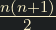for row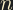.

### Lower bound

We can set a trivial lower bound for the solution at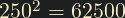. The square root of a given number will always be in the middle of the list of factors; either the middle term (if the number is a square), or in between the middle two terms. The smallest the middle term in a list of 500 terms can be is 250. We can plug this into the formula for the sum of natural numbers to get a trivial lower bound for.### Coprime integers

This problem can be broken down to make it simpler. Consider the following:

• Take a numberand choose any two factors
• List the factors of each factor
• Multiply each term from each list with each term from the other
• The resulting list will be all the factors of, including potential duplicates.

Lets try it with 72. Pick two random factors: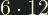. 6: 1, 2, 3, 6 12: 1, 2, 3, 6, 12 Perform what is essentially long multiplication Result: 1, 2, 3, 6, 12, 2, 4, 6, 12, 24, 3, 6, 9, 18, 36, 6, 12, 18, 72 Eliminate duplicates: 1, 2, 3, 4, 6, 9, 12, 18, 36, 72 Convince yourself that this works.

This is not especially useful in this scenario, we create a lot of work by having to eliminate all these duplicates. But if we choose two factors that are coprime, then by the fundamental theorem of arithmetic we are guaranteed to not have any duplicates.

We can therefore deduce that for coprime integers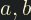: the number of divisors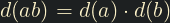.

### Combining with the sum of natural numbers

In the formula for for the sum of natural numbers:and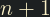—being consecutive numbers—must be coprime. Finding the factors of these two and multiplying will be faster than just factorising all triangular numbers. We have to deal with the pesky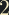on the denominator, but given that eitherandwill be even, we can just assign the 2 to the even one. The coprime nature will be preserved, as the only thing we've done is eliminate 2 as one of the factors.

### Further musings

I feel like there could still be a better way to solve this, by analysing which integers can appear in a factor list. I don't know much about this area of maths, but it strikes me that there's probably some rules around the spacing between factors of a number. For example, there is no integer with 10 divisors such that the first five are the consecutive integers 1,2,3,4,5.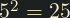, not divisible by 4.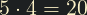, not divisible by 3. The only example I can think of that works is 12: 1, 2, 3, 4, 6, 12. Is this the highest? If not, why? I'm getting off track, but the point is that there must be rules to the spacing of factors; the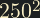lower bound is clearly too low. Could we construct enough rules to move the lower bound all the way up to the actual answer? I won't attempt this now, however.

## Solution

Implement a prime generator to be used for factorisation.

/* Generate a list of primes up to the upper bound
* primes should be a zero array of the correct length */
int gen_primes(int *primes, int upper_bound)
{
int prime_count = 0;
for (int i = 2; i < upper_bound; i++) {
/* Treat a 0 value as potentially prime */
if (primes[i] == 0) {
/* Stick all the actual primes at the beginning of the array */
primes[prime_count++] = i;
for (int j = i + i; j < upper_bound; j += i) {
/* Treat a -1 value as not prime */
primes[j] = -1;
}
}
}
return prime_count;
}


Implement a function to count the divisors of a given integer by multiplying the exponents of each prime factor, plus one. This website explains well how this works.

#include <math.h>
int get_num_factors(int *primes, int prime_count, int n)
{
int sqrt = (int)sqrtf(n);
int result = 1;
int i = 0, prime = 0;

/* Find all the primes below the square root */
while (prime < sqrt) {
prime = primes[i++];
int exponent = 0;
while (n % prime == 0) {
n = n / prime;
exponent++;
}
result *= (exponent + 1);

if (i >= prime_count) {
return -1;
}
}

/* There'll only ever be a maximum of one prime factor greater than 0
* If there is one for this number we need it to contribute to the total */
if (n > 1) {
result *= 2;
}

return result;
}


For each number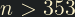, calculate the number of factors of. The computation forcan be reused forin the next loop.

#include <stdio.h>
#define TARGET 500
#define LOWER_BOUND_N 354
/* 10000 is just a reasonable seeming upper bound I chose for the primes */
#define UPPER_BOUND_PRIMES 10000

int main()
{
int primes[UPPER_BOUND_PRIMES];
int prime_count = gen_primes(primes, UPPER_BOUND_PRIMES);

int n = LOWER_BOUND_N;
int nd_triangle = 0;
int a = n % 2 == 0 ? n / 2 : n;
int nd_a = get_num_factors(primes, prime_count, a);
while (nd_triangle <= TARGET) {
int b = n % 2 == 0 ? n + 1 : (n + 1) / 2;
int nd_b = get_num_factors(primes, prime_count, b);

if (nd_b < 0) {
printf("Not enough primes to factorise %d!", b);
return 1;
}

nd_triangle = nd_a * nd_b;
nd_a = nd_b;
n++;
}
n--;
printf("%d", (n * (n + 1)) / 2);
}